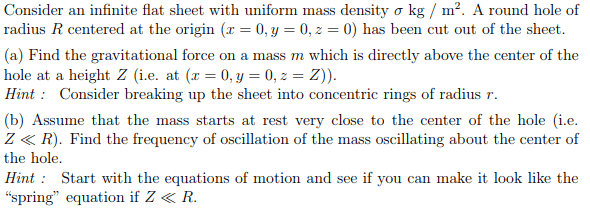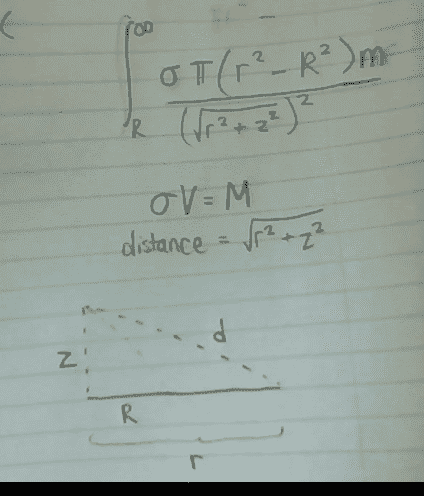# Finding the gravitational force over a flat infinite sheet

Phantoful

## Homework StatementF=ma
F=Gm1m2/r2
Gauss' Law?

## The Attempt at a Solution

I'm not sure if I should be using Gauss' Law for this question, because I've never heard of it or learned about it. I'm currently taking multi-variable calculus (gradients, vectors, etc.). From what I know, the gravitational force should be found using an integral from R to infinity for the area of the plane... but I'm not sure what to do from there.

G*∫((mσ)/(Z^2))*dA from R to ∞?

#### Attachments

Homework Helper
Gold Member
2022 Award
You can't "dA from R". Decide what your variable of integration is and use that in the range, in the expression for the force and in the expression for the infinitesimal element.

Phantoful
Okay, I'm starting to visualize it now, but I'm not sure how to put it into mathematics. I want to find the gravitational force for each concentric circle that has mass σπ(r2-R2) because V*σ=Msheet, and just using geometry the distance between the mass and the circles would be (r2+Z2)½, here's where I am:However, I know that it's not correct mathematically because I'm not sure how to include dr, which I know is supposed to be in here. How can I make it a part of my integral? Or am I approaching this incorrectly?

#### Attachments

grzz
The dr is included by putting the correct mass of the infinitesimal ring which is σ2πrdr.

grzz
Note also that only the vertical component of the force due to the ring is to be considered.

Phantoful
The dr is included by putting the correct mass of the infinitesimal ring which is σ2πrdr.
Note also that only the vertical component of the force due to the ring is to be considered.

I'm not sure how to visualize it this way, but what you're saying is that my equation (inside the integral) should be ((Gσ2πr*m)/Z2)*dr? I used the second equation from #2 in my original post, m being the mass of the point and G being the constant. Why is the equation for circumference instead of area used? I made the integral from R to ∞, which I believe is correct, right?